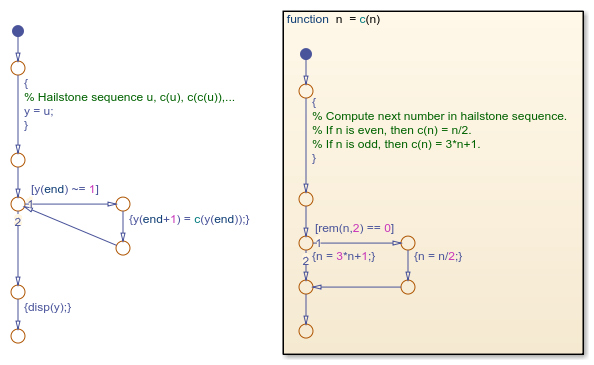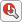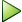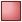## Convert MATLAB Code into Stateflow Flow Charts

To transform your MATLAB® code into Stateflow® flow charts and graphical functions, use the Pattern Wizard. Supported patterns for conversion include:

• `if`, `if-else`, and other nested decision statements.

• `for` and `while` loops.

• `switch` statements.

The Pattern Wizard can convert MATLAB functions and scripts.

• MATLAB functions are converted to Stateflow graphical functions.

• MATLAB scripts are converted to Stateflow flow charts.

Converting MATLAB code is supported only in standalone Stateflow charts. For more information, see Create Stateflow Charts for Execution as MATLAB Objects.

### Create Flow Charts from MATLAB Scripts

This MATLAB script empirically verifies one instance of the Collatz conjecture. When given the numeric input `u`, the script computes the hailstone sequence n0 = `u`, n1, n2, n3, ⋯ by iterating this rule:

• If ni is even, then ni+1 = ni/2.

• If ni is odd, then ni+1 = 3ni + 1.

The Collatz conjecture states that every positive integer has a hailstone sequence that eventually reaches one.

```% Hailstone sequence u, c(u), c(c(u)),... y = u; while y(end) ~= 1 y(end+1) = c(y(end)); end disp(y); function n = c(n) % Compute next number in hailstone sequence. % If n is even, then c(n) = n/2. % If n is odd, then c(n) = 3*n+1. if rem(n,2) == 0 n = n/2; else n = 3*n+1; end end```
The script executes a `while` loop that repeatedly calls the auxiliary function `c` until it produces an output value of one. The function `c` consists of a conditional `if`-`else` statement whose output depends on the parity of the input.

To convert this script into a flow chart and a graphical function:

1. Open a new standalone chart.

`edit hailstone.sfx`
2. On the State Chart tab, select > .

3. In the dialog box, choose the MATLAB script and click Open. The Pattern Wizard adds a flow chart and a graphical function to your Stateflow chart. Double-click the graphical function to see its contents.4. In the Symbols pane, click. The Stateflow Editor resolves `u` and `y` as local data.

6. To execute the chart from the Stateflow Editor, in the Symbols pane, enter a value of `u` = 9 and click. While the flow chart is executing, the Stateflow Editor highlights the active transitions through chart animation. When the execution stops, the MATLAB Command Window displays the hailstone sequence, starting with a value of nine:
``` 9 28 14 7 22 11 34 17 52 26 13 40 20 10 5 16 8 4 2 1 ```
7. Click.
Suppose that you use `nargin` in a MATLAB function that you convert to a graphical function in a chart. Because `nargin` counts the chart object as one of the input arguments of the graphical function, the value of `nargin` in the graphical function is equal to one plus the value of `nargin` in the original MATLAB function. For more information, see Execute a Standalone Chart.